Magnetic Field Formulas

(Direct from Maxwell)These three simple formulas can be derived directly from Maxwell's fourth equation, or Ampere's Law. Magnetic field due to an infinite, straight current filament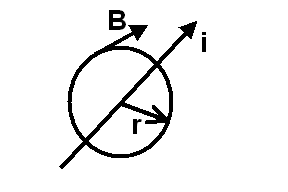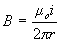B is the magnetic field, in teslas. The direction of the field is tangent to a circle on a radius r (in meters) from the wire.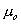is the permeability constant (1.26x10-6 H/m) i is the current in the wire, in amperes.Field inside a straight, infinite, air core solenoid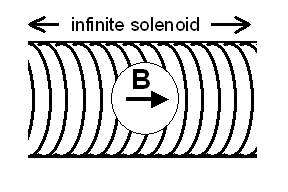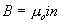B is the magnetic field inside the solenoid, in teslas. The direction of the field is parallel to the axis of the solenoid. There is no field outside the solenoid.is the permeability constant (1.26x10-6 H/m) i is the current in the wire, in amperes. n is the number of turns of wire per unit length of the solenoid, in 1/meters.Field inside an air core toroid coil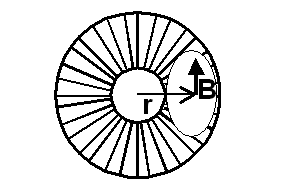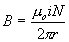B is the magnetic field, in teslas. The direction of the field is tangent to a circle on a radius r (in meters) from the center of the toroid.is the permeability constant (1.26x10-6 H/m) i is the current in the wire, in amperes. N is the total number of turns of wire in the toroid.